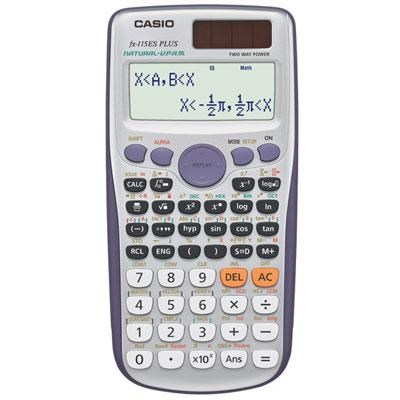Home  >  Calculators & Accessories >  Item # CASFX115ESPLUS (MFR# FX-115ESPLUS)x<>
+       -

# Casio Scientific 417 Function Calculator - FX-115ESPLUS

Not Available.
Condition: Brand new, USA Warranty
Casio Advanced Scientific Calculator with Natural Textbook Display format: 417 Functions, Fraction calculation, Combination & Permutation, Logarithm log, List STAT data editor, Std deviation. Comes with slide on hard caseCasio's latest and most advanced scientific calculator features new Natural Textbook Display and improved math functionality. FX-115ES PLUS has been designed to be the perfect choice for high school and college students learning General Math; Trigonometry; Statistics; Algebra I and II; Calculus; Engineering; Physics.

Product Specifications:

General

• Power Supply: Solar +(G13/LR44)x1
• Display (Lines or Dots): 16 x 4 Lines (96 x 31 Dots)
• Auto Power OFF: Yes

Display / Expression

• Natural Textbook Display: Yes
• Input: Yes
• Output (Fraction): Yes
• Output (pi; root): Yes
• Transformations: Sexagesimal-Decimal Transformation: Yes; Engineering Notation: Yes; Recurring Decimal: Yes

Basic Functions

• Trigonometric; Inverse Trigonometric: Yes
• Hyperbolic; Inverse Hyperbolic: Yes
• Exponental; Logarithmic (log;ln;10x;ex): Yes
• Power and Radical Root: Yes
• Fraction: Yes
• Mixed Fraction: Yes
• Pi: Yes
• Rounding: Yes
• Angle Unit / Conversion (Deg; Rad; Grad): Yes
• GCD/LCM: Yes
• Remainder (R?): Yes
• Base Specified Logarithmic (Log aB): Yes
• Prime Factorization: Yes

Utilities

• Variables: 9
• Answer Memory (Ans): Yes
• Previous Answer Memory (Pre Ans): Yes
• Solve function: Yes
• CALC Function (Stored FormulaCalculation): Yes
• Scientific Constant: 40
• Metric Conversion: 40

Special Features

• Statistics: Yes
• Standard Deviation: Yes
• Regression: Yes
• Combination; Permutation (nCr; nPr): Yes
• Random Numbers: Yes
• Random Integers (RanInt): Yes
• Integer / Nearest Lower Integer (Int;Intg): Yes
• Table Function: Yes
• Type of Table: f(x) f(x); g(x)
• Complex Number Calculation: Yes
• Differentiation; Integration Calculation: Yes
• Base-N Calculations: Yes
• Matrix Calculations: Yes
• Vector Calculations: Yes
• Equation Calculation: Yes
• Simultaneous Equation: Yes
• Polynomial Equation: Yes
• Inequality Calculation: Yes
• Sum Calculation (SIGMA): Yes
• Product Calculation (Product of f(x)): Yes
• Verify Function: Yes
Sign up for coupons and special offers
My Account
Customer Service
Contact Us
F.A.Q.
PoliciesMobile Website
Coupons
Shop By Brand
New Releases
RebatesReview Us
Testimonials
About Us
Our Blog© 1996-2019   beachcamera.com.  All rights reserved.
beachcamera.com is a registered trademark.